Courses

# Dimensional Analysis Civil Engineering (CE) Notes | EduRev

## Civil Engineering (CE) : Dimensional Analysis Civil Engineering (CE) Notes | EduRev

The document Dimensional Analysis Civil Engineering (CE) Notes | EduRev is a part of the Civil Engineering (CE) Course Civil Engineering SSC JE (Technical).
All you need of Civil Engineering (CE) at this link: Civil Engineering (CE)

Chapter 8 Dimensional Analysis

•  Velocity potential = [L2 T–1]
Stream function = [L2 T–1]
Acceleration = [LT–2]
Vorticity = [T–1] 
• Total no. of variables influencing the problem is equal to the no. of independent variables plus one, one being the no. of dependent variable. 
• Buckingham p theorem states that if all the n-variable are described by m fundamental dimensions, they may be grouped into (n - m) dimensions p terms. 
• Selection of 3 repeating variables from the geometry of flow, fluid properties and fluid motion. 
• Geometric similarity - similarity of shape
Kinematic similarity - similarity of motion
dynamic similarity - similarity of forces
 Number Equation Significance Reynolds No.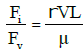Flow in closed conduit pipe Froude No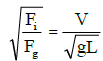where a free surface is present,structure eg. weirs spillway, channels, etc. w here gravity force is predominant. Eulers No.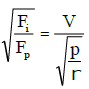In cavitation studies. Mach No.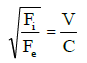where fluid compressibility is important. Weber No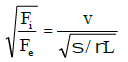In capillary studies.

Reynolds Model Law :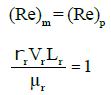(i) Velocity ratio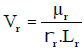(ii) Time ratio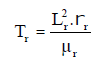(iii) Acceleration ratio,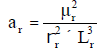(iv) Force ratio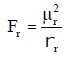(v) Power ratio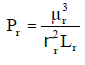(vi) Discharge ratio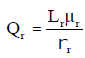Applications of Reynold’s Model Law :-

• Flow through small sized pipes 
• Low velocity motion around automobiles and aeroplane. 
• Submarines completely under water. 
• Flow through low speed trubo machines. 

• Froude’s Model law :

(Fr) m = (Fr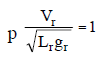(i) Time ratio
Tr = Lr1/2 gr–1/2 = Lr1/2

(ii) Acceleration ratio ar = gr = 1

(iii) Force ratio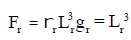(iv) Power ratio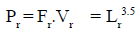(v) Discharge ratio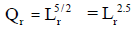Applications : 

• Open channels 
• Notches & weirs 
• Spill ways & dams 
• Liquid jets from orifice 
• Ship partially submerged in rough & turbulent sea
Offer running on EduRev: Apply code STAYHOME200 to get INR 200 off on our premium plan EduRev Infinity!

## Civil Engineering SSC JE (Technical)

109 docs|50 tests

,

,

,

,

,

,

,

,

,

,

,

,

,

,

,

,

,

,

,

,

,

;